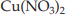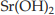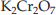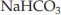×
Get Full Access to Introductory Chemistry - 5 Edition - Chapter 16 - Problem 55p
Get Full Access to Introductory Chemistry - 5 Edition - Chapter 16 - Problem 55p

×

# Assign an oxidation state to each element in eachISBN: 9780321910295 34

## Solution for problem 55P Chapter 16

Introductory Chemistry | 5th Edition

• Textbook Solutions
• 2901 Step-by-step solutions solved by professors and subject experts
• Get 24/7 help from StudySoup virtual teaching assistantsIntroductory Chemistry | 5th Edition

4 5 1 342 Reviews
27
4
Problem 55P

Problem 55P

Assign an oxidation state to each element in each compound.

(a) Cu(NO3)2

(b) Sr(OH)2

(c) K2Cr2O7

(d) NaHCO3

Step-by-Step Solution:

Problem 55P

Assign an oxidation state to each element in each compound.

(a)(b)(c)(d)Step by Step Solution

Step 1 of 5

Redox is formed from two words that are reduction and oxidation. The redox reaction is defined as the reaction in which both oxidation and reduction occur in a single reaction. Redox reactions are significant reactions in chemistry. The oxidation states of electropositive and electronegative atoms are generally fixed.

Step 2 of 5

Step 3 of 5

##### ISBN: 9780321910295

This full solution covers the following key subjects: assign, compound, element, nahco, oxidation. This expansive textbook survival guide covers 19 chapters, and 2046 solutions. The answer to “Assign an oxidation state to each element in each compound.(a) Cu(NO3)2(b) Sr(OH)2(c) K2Cr2O7(d) NaHCO3” is broken down into a number of easy to follow steps, and 14 words. The full step-by-step solution to problem: 55P from chapter: 16 was answered by , our top Chemistry solution expert on 05/06/17, 06:45PM. Since the solution to 55P from 16 chapter was answered, more than 378 students have viewed the full step-by-step answer. This textbook survival guide was created for the textbook: Introductory Chemistry, edition: 5. Introductory Chemistry was written by and is associated to the ISBN: 9780321910295.

## Discover and learn what students are asking

Statistics: Informed Decisions Using Data : Discrete Random Variables
?What is a random variable?

Unlock Textbook Solution